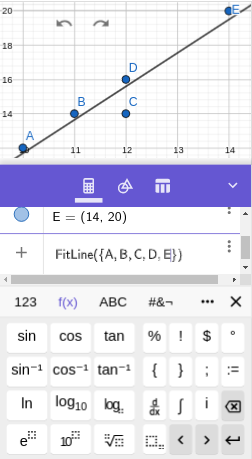# Intermediate Algebra: Functions and Graphs

## SectionA.5Regression

Make a scatterplot for the points $$(10,12)\text{,}$$ $$(11,14)\text{,}$$ $$(12,14)\text{,}$$ $$(12,16)\text{,}$$ $$(14,20)\text{.}$$ Then find the equation of the lease-squares regression line and plot it on top of the scatterplot.
We enter each of the five ordered pairs. The output history will show each labeled with a capital letter, such as $$A=(10,12)\text{.}$$